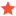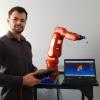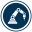How to rotate object at the edge of conveyor?Rev123Junior MemberPosts: 2 Threads: 1 Joined: Nov 2020 Reputation: 0 03-05-2021, 09:26 AM Hi all Good Morning :),  So i've been doing my conveyor project using RunConveyor script from "Example-06.e-Conveyor with 2 UR robots" on the library. The object is moving but i want the object to rotate Y=-45 degrees when the object reach the edge.  I have been editing the section where "objects that reached the end of the conveyor and were not picked" to ([0,0,0,0,-45,0]) but it turns out the object won't rotate.  This is the script: CONVEYOR_NAME = 'ConvBI' PICKABLE_OBJECTS_KEYWORD = 'Bundle' # Speed of movement in MM/S with respect to the conveyor coordinates: MOVE_SPEED_MMS = [0,50,0] REFRESH_RATE = 0.005 # Define workspace of the conveyor to pick the objects: CONV_SZ_X_MIN = 0 CONV_SZ_X_MAX = 440 CONV_SZ_Y_MIN = 0 CONV_SZ_Y_MAX = 990 CONV_SZ_Z_MIN = -200 CONV_SZ_Z_MAX = +500 # Move objects that reached the end of the conveyor and were not picked: FALLEN_OBJECTS = ([0,0,0,0,-45,0]) # Get the conveyor item and reference for work space: conv = RDK.Item(CONVEYOR_NAME) conv_reference = conv.Parent() poseconv = conv_reference.PoseAbs() # One second in real life means 1 second of simulation. The simulation speed is taken from the station SIMULATION_SPEED = 1 def is_inside_conveyor(pose):     """Checks if a pose is inside the conveyor workspace"""     pos = pose.Pos()     if pos > CONV_SZ_X_MIN and pos < CONV_SZ_X_MAX and pos > CONV_SZ_Y_MIN and pos < CONV_SZ_Y_MAX and pos > CONV_SZ_Z_MIN and pos < CONV_SZ_Z_MAX:         return True     return False def conveyor_move_object(pose, delta_time):     """Moves the object pose through the conveyor depending on the time and speed"""     delta_mm = mult3(MOVE_SPEED_MMS, delta_time)     newpose = transl(delta_mm)*pose     return newpose # Get all objects (string list) all_objects = RDK.ItemList(ITEM_TYPE_OBJECT, True) # Convert object list into item pointers (faster) # Also filter the list to take into account pickable objects only objects = [] objects_name = [] objects_active = [] for i in range(len(all_objects)):     if all_objects[i].count(PICKABLE_OBJECTS_KEYWORD) > 0:         objects.append(RDK.Item(all_objects[i]))         objects_name.append(all_objects[i])                 objects_active.append(False) # The number of objects that can go in the conveyor nobjects = len(objects) # Infinite loop to simulate the conveyor behavior current_time = 0 tic() time_last = toc() while True:     # First: Look for objects that are not in the conveyor but are in the conveyor workspace     for i in range(nobjects):         obj_i = objects[i]                  # Skip if the object is already in the conveyor         if objects_active[i]:             continue                  # Check if the object has already been taken by a tool. If so, do not take it in the conveyor         if obj_i.Parent().Type() == ITEM_TYPE_TOOL:             continue         # Check if the object is within the conveyor work area         posei = obj_i.PoseAbs()         poseirel = invH(poseconv)*posei         if is_inside_conveyor(poseirel):             # take the object             obj_i.setParentStatic(conv)             print('Adding object %s to the conveyor' % objects_name[i])             objects_active[i] = True     # Second step: Update the position of every object in the conveyor     SIMULATION_SPEED = RDK.SimulationSpeed()     time_current = toc()     time_delta = time_current - time_last     time_last = time_current     current_time = current_time + time_delta*SIMULATION_SPEED     # Make a list of objects with their matching positions to update     obj_items = []     obj_poses_abs = []     for i in range(nobjects):         obj_i = objects[i]         # Check if the object has been picked from the conveyor         if objects_active[i] and not obj_i.Parent().equals(conv):             objects_active[i] = False             print('Object %s was picked from the conveyor' % objects_name[i])             continue         # Skip update for objects that are not in the conveyor         if not objects_active[i]:             continue         # Update the position of the object         posei = invH(poseconv)*obj_i.PoseAbs()         newposei = conveyor_move_object(posei, time_delta*SIMULATION_SPEED)         if not is_inside_conveyor(newposei):             print('Warning!! Object %s fell from the conveyor at %.1f seconds after simulation started' % (objects_name[i], current_time))             #raise Exception('Object %s was not picked from the conveyor!' % objects_name[i])             newposei = transl(FALLEN_OBJECTS)*newposei             objects_active[i] = False                  #obj_i.setPose(newposei) # this will provoke a refresh (can be slow)         obj_items.append(obj_i)         obj_poses_abs.append(poseconv*newposei)     # Update the object positions     RDK.setPosesAbs(obj_items, obj_poses_abs)     # Take a break...     pause(REFRESH_RATE) so, this is the result that i get now:  https://ibb.co/rbv3CHJ and also this is my expected result:  https://ibb.co/m986pwm i hope somebody will help me to solve this. Thanks in advance. Rev. Find ReplyOlivierModeratorPosts: 138 Threads: 0 Joined: Aug 2020 Reputation: 16 03-05-2021, 03:19 PM (This post was last modified: 03-05-2021, 03:40 PM by Olivier.) Hi Rev, Take a look at this example. If that doesnt help, maybe you can share the station with me so I can have a closer look at it? Olivier Attached FilesConveyor example.rdk (Size: 151.47 KB / Downloads: 240) Find ReplyRev123Junior MemberPosts: 2 Threads: 1 Joined: Nov 2020 Reputation: 0 03-10-2021, 07:08 AM (03-05-2021, 03:19 PM)Olivier Wrote: Hi Rev, Take a look at this example. If that doesnt help, maybe you can share the station with me so I can have a closer look at it? Olivier Hi Olivier, Thank you for your help and sorry for the late response, i will try your example and also inform you my progress. Rev Find Reply